## 8-4 Dynamic Time Warping

[english][all]

(請注意：中文版本並未隨英文版本同步更新！)

DTW 是 Dynamic Time Warping 的簡稱，中文可以翻譯成「動態時間扭曲」或是「動態時間校正」，這是一套根基於「動態規劃」（Dynamic Programming，簡稱 DP）的方法，可以有效地將搜尋比對的時間大幅降低。

• 端點關係：(p1, q1) = (1, 1), (pk, qk) = (m, n)。此端點關係代表這是「頭對頭、尾對尾」的比對。
• 局部關係：假設最佳路徑上任一點可以表示成 (i, j)，那麼其前一點路徑只有三種可能：(i-1, j), (i, j-1), (i-1, j-1)。此局部關係定義了路徑的連續性，而且也規定了 t 的任一個元素至少對應一個 r 的元素，反之亦然。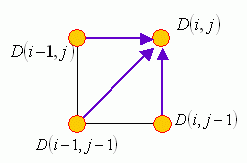1. 目標函數之定義：定義 D(i, j) 是 t(1:i) 和 r(1:j) 之間的 DTW 距離，對應的最佳路徑是由 (1, 1) 走到 (i, j)。
2. 目標函數之遞迴關係：D(i, j) = ∣t(i) - r(j)∣ + min{D(i-1, j), D(i-1, j-1), D(i, j-1)}，其端點條件為 D(1, 1) = ∣t(1) - r(1)∣
3. 最後答案：$D(m, n)$.

1. 目標函數：定義 D(i, j) 是 t(i:m) 和 r(j:n) 之間的 DTW 距離，對應的最佳路徑是由 (i, j) 走到 (m, n)。
2. 遞迴關係：D(i, j) = ∣t(i) - r(j)∣ + min{D(i+1, j), D(i+1, j+1), D(i, j+1)}，端點條件為 D(m, n) = ∣t(m) - r(n)∣
3. 最後答案：D(1, 1)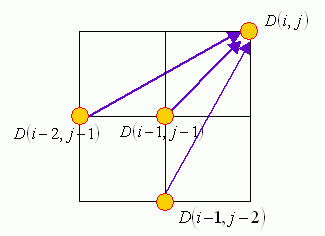• 會「跳點」，因此若有一點雜訊，最佳的 DTW 路徑將會跳掉此點。
• 如果最佳路徑是對應於總距離的最小值，那麼最佳路徑會盡量走 27 或 63 度，以使對應到的點數降低。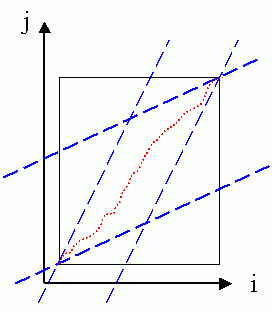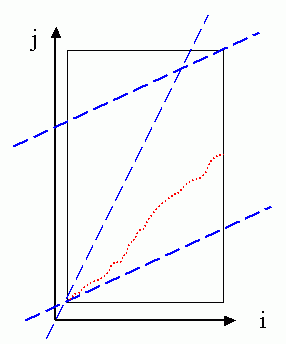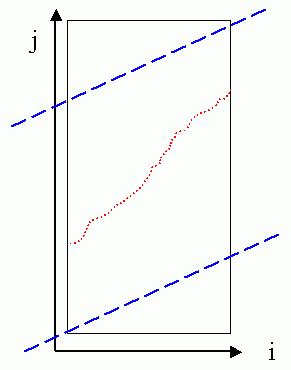It should be noted that the global path constraints are induced as a result of the local path constraints of 27°-45°-63°. However, when we are using the local path constraints of 0°-45°-90°，sometimes we still apply the global path constraints in order to limit the mapping path to a reasonable shape. In summary, the use of global path constraints serves two purposes:

• Limit the mapping path to be a reasonable one.

In the following, we shall give some examples of DTW. For simplicity, we shall distinguish two types of DTW:

• Type-1 DTW uses 27°-45°-63° local path constraint.
• Type-2 DTW uses 0°-45°-90° local path constraint.

First, we can use dtw.m (with dtwOpt.type=1) and dtwPathPlot.m to plot the mapping path of type-1 DTW in the following example:

Example 1: dtw1Plot01.mvec1=[71 73 75 80 80 80 78 76 75 73 71 71 71 73 75 76 76 68 76 76 75 73 71 70 70 69 68 68 72 74 78 79 80 80 78]; vec2=[69 69 73 75 79 80 79 78 76 73 72 71 70 70 69 69 69 71 73 75 76 76 76 76 76 75 73 71 70 70 71 73 75 80 80 80 78]; dtwOpt=dtwOptSet; dtwOpt.type=1; [minDist, dtwPath, dtwTable] = dtw(vec1, vec2, dtwOpt); dtwPathPlot(vec1, vec2, dtwPath);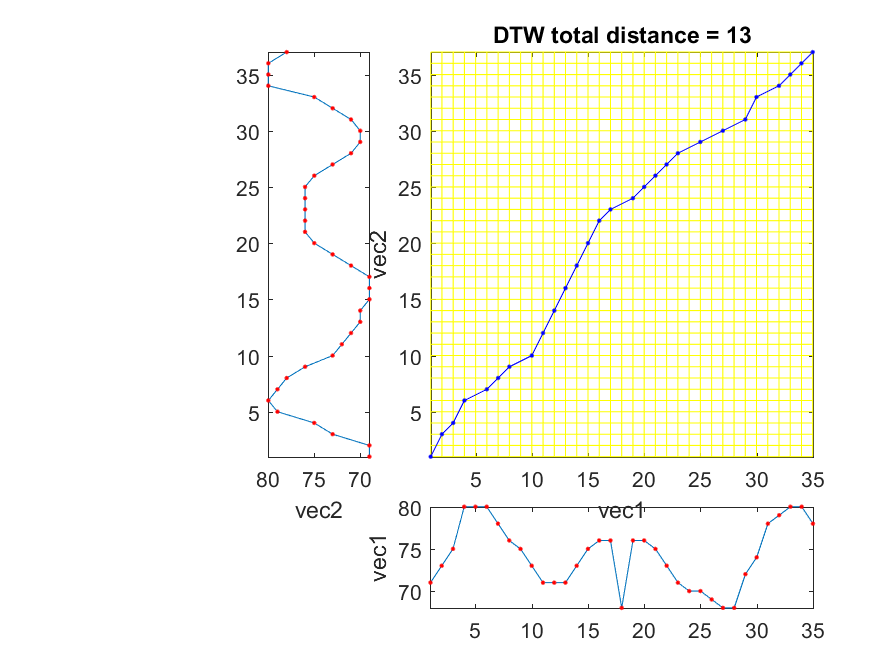In the above example, we deliberately put an outliner in vec1. Due to the local path constraints of type-1 DTW, this outliner is skipped in the optimum mapping path.

Similarly, we can use dtw.m (with dtwOpt.type=2) and dtwPathPlot.m to plot the mapping path of type-2 DTW:

Example 2: dtw2Plot01.mvec1=[71 73 75 80 80 80 78 76 75 73 71 71 71 73 75 76 76 68 76 76 75 73 71 70 70 69 68 68 72 74 78 79 80 80 78]; vec2=[69 69 73 75 79 80 79 78 76 73 72 71 70 70 69 69 69 71 73 75 76 76 76 76 76 75 73 71 70 70 71 73 75 80 80 80 78]; dtwOpt=dtwOptSet; dtwOpt.type=2; [minDist, dtwPath, dtwTable] = dtw(vec1, vec2, dtwOpt); dtwPathPlot(vec1, vec2, dtwPath);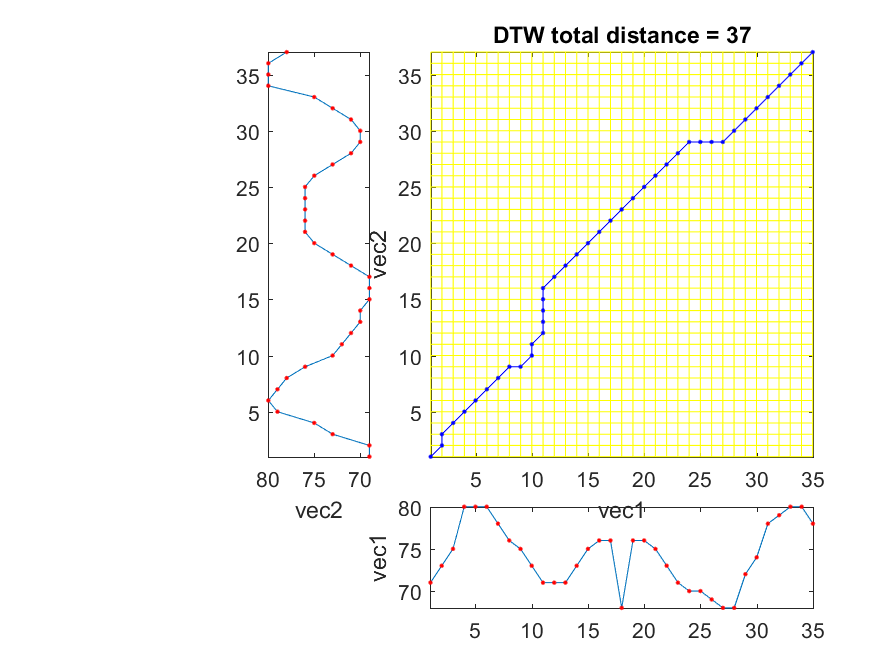The outliner cannot be skipped since the local path constraints require each point in a vector has at least one correspondence in the other vector.

By using dtwBridgePlot.m, we can use red lines to connect corresponding points in two vectors, as follows:

Example 3: dtwBridgePlot02.mvec1=[71 73 75 80 80 80 78 76 75 73 71 71 71 73 75 76 76 68 76 76 75 73 71 70 70 69 68 68 72 74 78 79 80 80 78]; vec2=[69 69 73 75 79 80 79 78 76 73 72 71 70 70 69 69 69 71 73 75 76 76 76 76 76 75 73 71 70 70 71 73 75 80 80 80 78]; dtwOpt=dtwOptSet; dtwOpt.type=1; [minDist1, dtwPath1, dtwTable1] = dtw(vec1, vec2, dtwOpt); dtwOpt.type=2; [minDist2, dtwPath2, dtwTable2] = dtw(vec1, vec2, dtwOpt); subplot(2,1,1); dtwBridgePlot(vec1, vec2, dtwPath1); title('DTW alignment by type-1 DTW'); subplot(2,1,2); dtwBridgePlot(vec1, vec2, dtwPath2); title('DTW alignment by type-2 DTW');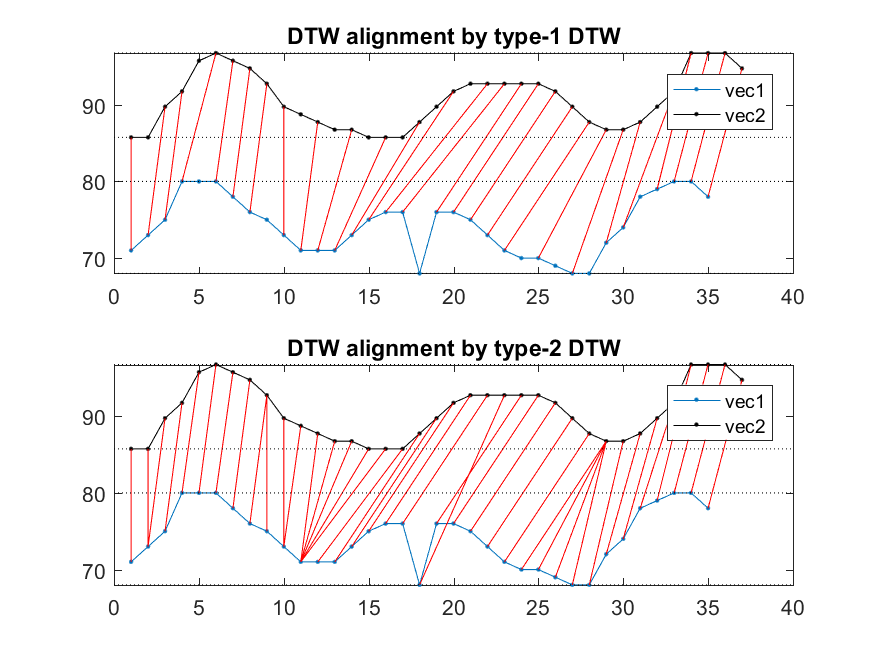It becomes more obvious that type-1 DTW can have empty correspondence while type-2 DTW can have multiple correspondences. Moreover, we can use an extra argument for dtwBridgePlot.m to put the two vectors in 3D space to have a more interesting representation:

Example 4: dtwBridgePlot03.mvec1=[71 73 75 80 80 80 78 76 75 73 71 71 71 73 75 76 76 68 76 76 75 73 71 70 70 69 68 68 72 74 78 79 80 80 78]; vec2=[69 69 73 75 79 80 79 78 76 73 72 71 70 70 69 69 69 71 73 75 76 76 76 76 76 75 73 71 70 70 71 73 75 80 80 80 78]; dtwOpt=dtwOptSet; dtwOpt.type=1; [minDist1, dtwPath1, dtwTable1] = dtw(vec1, vec2, dtwOpt); dtwOpt.type=2; [minDist2, dtwPath2, dtwTable2] = dtw(vec1, vec2, dtwOpt); subplot(2,1,1); dtwBridgePlot(vec1, vec2, dtwPath1, '3d'); title('DTW alignment by type-1 DTW'); view(-10, 70); subplot(2,1,2); dtwBridgePlot(vec1, vec2, dtwPath2, '3d'); title('DTW alignment by type-2 DTW'); view(-10, 70);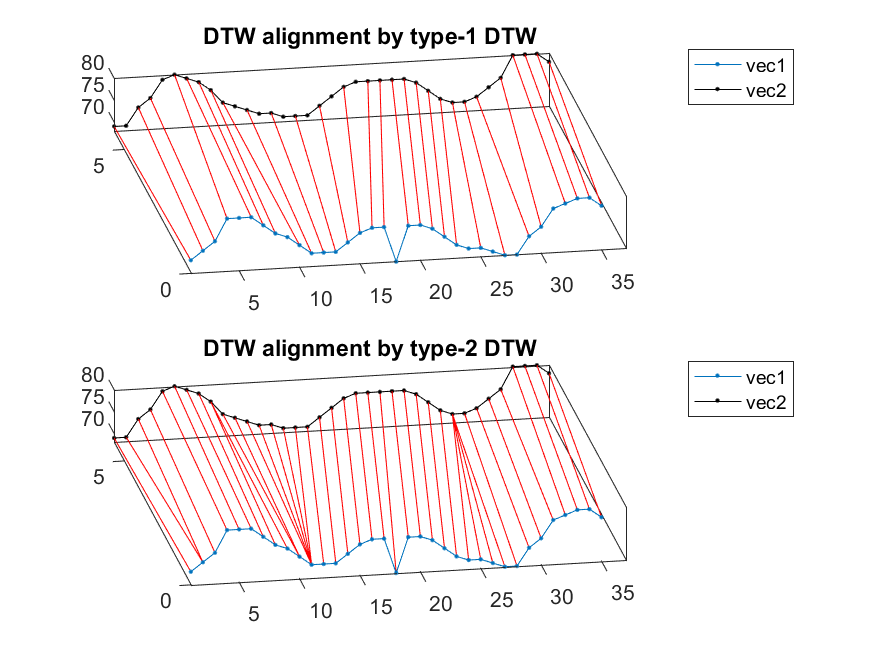Data Clustering and Pattern Recognition (資料分群與樣式辨認)